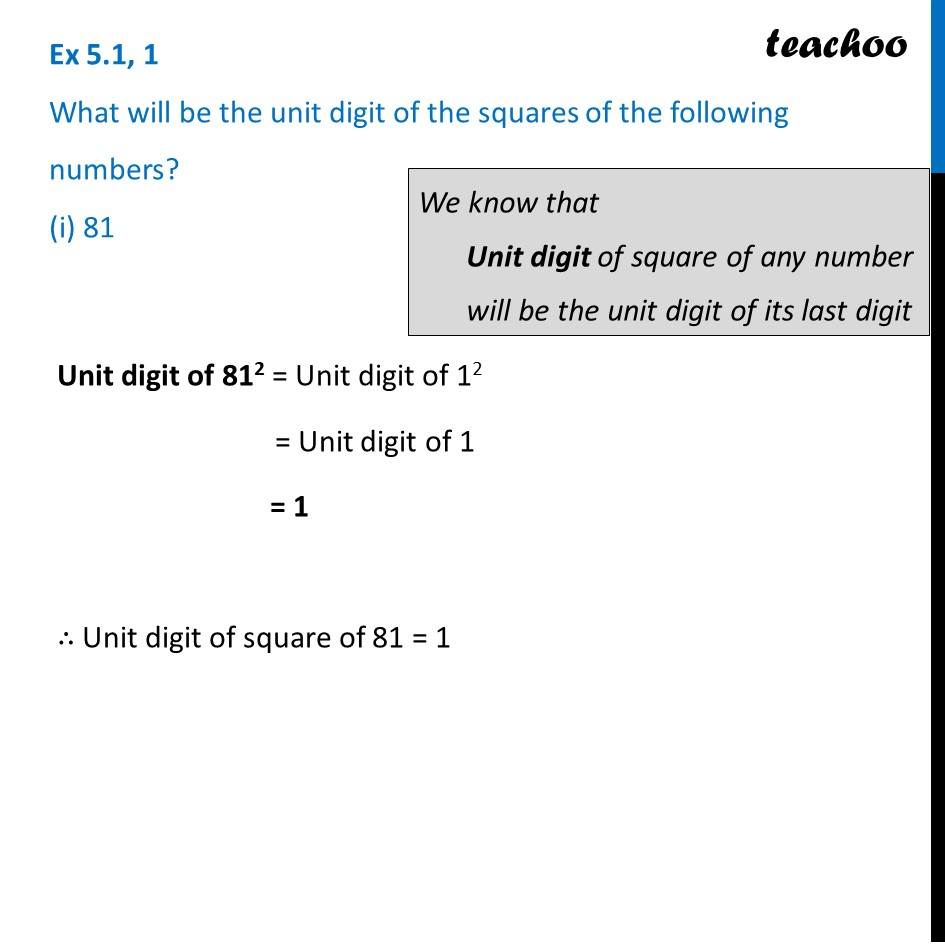Properties of square numbers

Chapter 5 Class 8 Squares and Square Roots
Concept wiseLearn in your speed, with individual attention - Teachoo Maths 1-on-1 Class

### Transcript

Ex 5.1, 1 What will be the unit digit of the squares of the following numbers? (i) 81 Unit digit of 812 = Unit digit of 12 = Unit digit of 1 = 1 ∴ Unit digit of square of 81 = 1